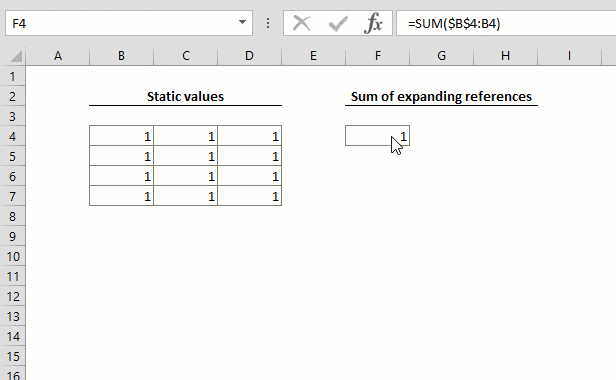An expanding reference or range is essentially a dynamic reference which can expand as a formula is copied down or across cells. In this article, we’re going to show you the expanding references in Excel and go over some common use cases.

# Creating an expanding reference

To create an expanding range, you need to use an absolute and a relative reference. The absolute reference works as an anchor while the relative cell is going to be updated as you copy the formula.

For example, assume the cell F4 has the following formula:

=SUM(\$B\$4:B4)

The reference in the formula points to the cell B4. When you copy it through to cell F7, the target range will become:

=SUM(\$B\$4:D7)

While the absolute B4 reference remains unchanged, the relative B4 reference becomes D7 after 3 rows and 2 columns. This is how you can establish an expanding range.# Use cases

You can use an expanding range in Excel in various ways. The most common use case is for calculating running counts and totals. An expanding range in a SUM or COUNT formula will help you evaluate values from the first cell, to the formula. For more information about the SUM and COUNT functions see:

How to calculate running totals with SUM

How to calculate running count

Another common use case is creating number series with the ROWS and COLUMNS functions. For more information about these two functions see:

ROWS

COLUMNS

Some formulas can be combined with expanding references to find unique items or merge columns. For more information about these use cases see:

How to find the unique items in a list

How to merge columns using formulas